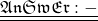## Assume that y varies directly with x. If y = -10 when x = -5, find y when x = 1. Write and solve a direct variation equation to find t

Question

Assume that y varies directly with x. If y = -10 when x = -5, find y when x = 1.
Write and solve a direct variation equation to find the answer.

in progress 0
4 weeks 2021-08-18T10:59:24+00:00 1 Answers 1 views 0

1.Actually Welcome to the concept of linear equation.

According to the relation when y=-10 , x =-5

we get the relation as,

===> y = 2x

hence, when x =1 ,

y = 2(1)

hence, y = 1